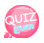# Ternary operator

The functionality is similar to the if else statement.
It is composed of three terms and is called ternary operator.

## How to Use the Ternary Operator

`((Condition) ? Execute if condition is true : Execute if condition is false )`

Isn't it hard to see how to use the above?
For example:

`\$number = ((1 == 1) ? 100 : 50)`

In the example above, the condition of the ternary operator is 1 == 1. Of course 1 equals 1, so the condition is true.
If true, there is 100. Therefore, 100 is assigned to the value of the variable \$number.
For example:

`\$number = ((1 == 2) ? 100 : 50)`

In the example above, the condition of the ternary operator is 1 == 2. Of course 1 is not 2, so the condition is false.
If it is false, there is 50. So 50 is assigned to the value of the variable \$number.
Let's find out more with the following example.

```<?php
for(\$i = 0; \$i <= 10; \$i++){
\$oddEven = ((\$i % 2 == 0) ? 'even' : 'odd');
echo "{\$i}is {\$oddEven}. <br>";
}
?>
```Yes Use it in this case. It's convenient because I don't write if else complicatedly. ^^